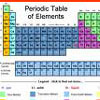You may also likeA Method of Defining Coefficients in the Equations of Chemical Reactions

A simple method of defining the coefficients in the equations of chemical reactions with the help of a system of linear algebraic equations.Tournament Scheduling

Scheduling games is a little more challenging than one might desire. Here are some tournament formats that sport schedulers use.Far Horizon

An observer is on top of a lighthouse. How far from the foot of the lighthouse is the horizon that the observer can see?

Carbon Footprints

Age 14 to 16 Challenge Level:

Masood, Akeel, and Dolapo from Wilson's School sent us their analysis:

An efficient small car produces about 120g per km of CO2.
An empty bus emits seven times more CO2 than a car per km.
So taking this into account, an empty bus emits 840g per km of CO2.
On average a normal commuter would travel 5km to get to work. So an efficient small car would emit 600g of CO2 when a bus would emit about 4200g. However there are more than seven people using a bus so altogether a bus is better than a car to travel to work.

If you were somehow able to travel to New York by train, you would be travelling 2000 miles.
On average, rail journeys produce 0.0602 kg of CO2 per passenger km
2000 miles is approximately 3200km.
So travelling to New York by train would emit $0.0602 \times 3200 = 192.64$ kg of CO2 for one person.
Travelling by plane, the figure given is 760.22kg of CO2 per person for a round trip, which is 380.11kg of CO2 each way, so travelling by train would be better if it were possible.